• C语言总结第七章、数组一维数组一维数组的定义一维数组的引用一维数组的初始化程序举例二维数组及多维数组二维数组的定义二维数组元素的引用二维数组元素的初始化程序举例字符数组和字符串字符数组 第七章、数组 ...
C语言总结第七章、数组一维数组一维数组的定义一维数组的引用一维数组的初始化程序举例二维数组及多维数组二维数组的定义二维数组元素的引用二维数组元素的初始化程序举例字符数组和字符串字符数组字符串
第七章、数组
数组是构造数据类型之一

数组： 有序数据的集合,用数组名标识
元素： 属同一数据类型,用数组名和下标确定

一维数组
一维数组的定义
定义方式：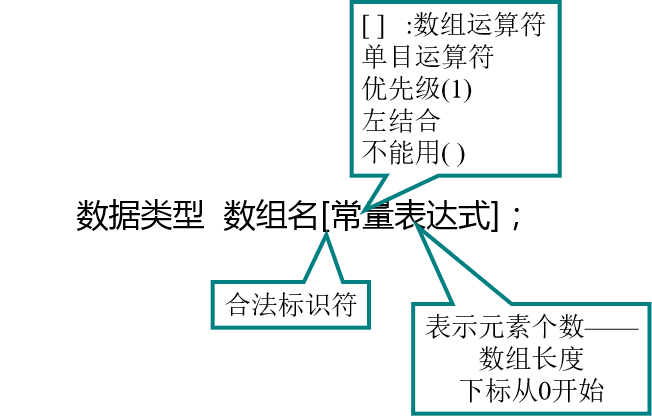例： int a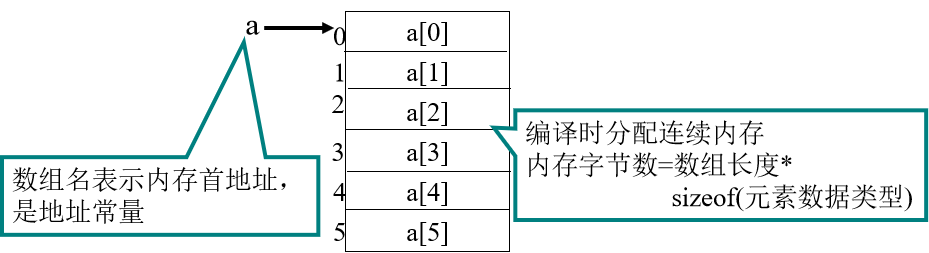一维数组的引用
1、数组必须先定义，后使用。
2、只能逐个引用数组元素，不能一次引用整个数组。
3、数组元素表示形式： 数组名[下标] ,下标可以是常量或整型表达式
。
一维数组的初始化
初始化方式：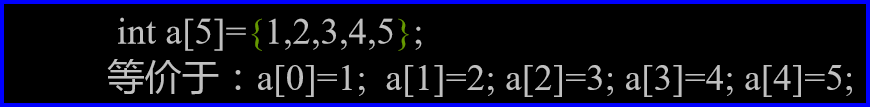说明：
1、数组不初始化，其元素值为随机数。
2、对static数组元素不赋初值，系统会自动赋以0值。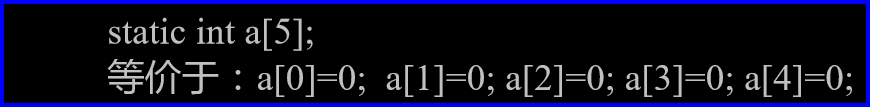3、只给部分数组元素赋初值。4、当全部数组元素赋初值时，可不指定数组长度。程序举例
例1： 读10个整数存入数组，找出其中最大值和最小值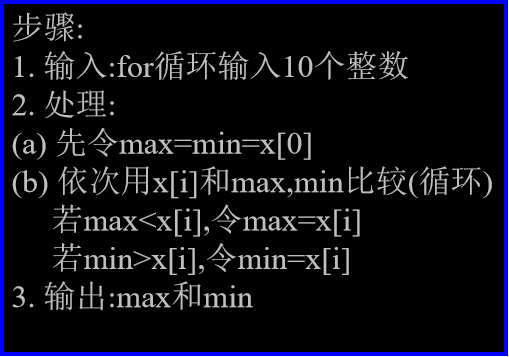/*示例1*/
#include <stdio.h>
#define SIZE 10
main()
{   int x[SIZE],i,max,min;
printf("Enter 10 integers:\n");
for(i=0;i<SIZE;i++)
{   printf("%d:",i+1);
scanf("%d",&x[i]);
}
max=min=x;
for(i=1;i<SIZE;i++)
{  if(max<x[i])  max=x[i];
if(min>x[i])  min=x[i];
}
printf("Maximum value is %d\n",max);
printf("Minimum value is %d\n",min);
}

例2： 用数组求Fibonacci数列前20个数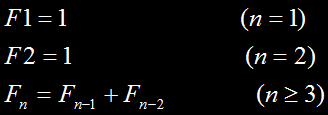/*示例2*/
#include <stdio.h>
main()
{   int i;
int f={1,1};
for(i=2;i<20;i++)
f[i]=f[i-2]+f[i-1];
for(i=0;i<20;i++)
{   if(i%5==0)  printf("\n");
printf("%12d",f[i]);
}
}

例3： 用冒泡法对10个数排序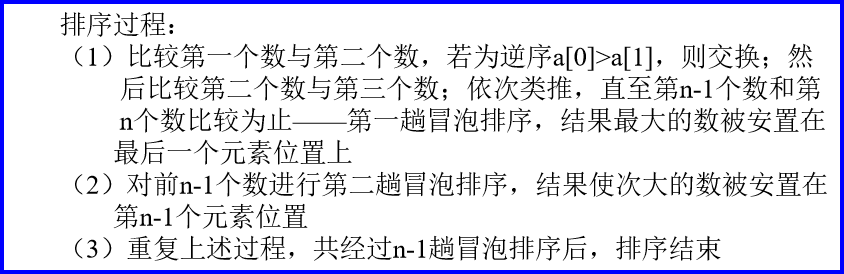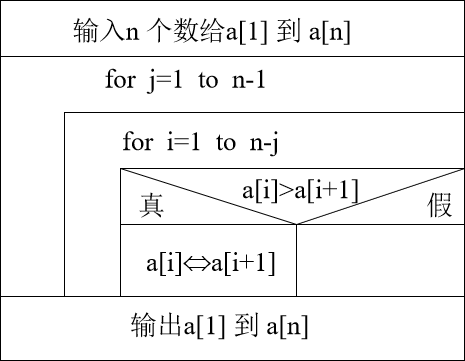/*示例3*/
#include <stdio.h>
main()
{   int a,i,j,t;
printf("Input 10 numbers:\n");
for(i=1;i<11;i++)
scanf("%d",&a[i]);
printf("\n");
for(j=1;j<=9;j++)
for(i=1;i<=10-j;i++)
if(a[i]>a[i+1])
{t=a[i]; a[i]=a[i+1]; a[i+1]=t;}
printf("The sorted numbers:\n");
for(i=1;i<11;i++)
printf("%d ",a[i]);
}

例4： 用简单选择法对10个数排序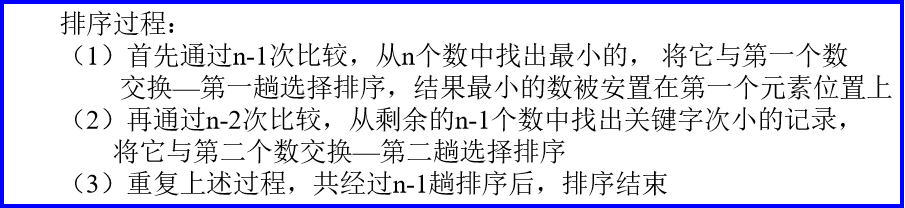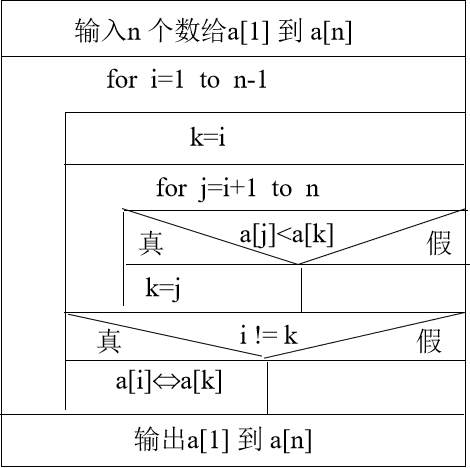/*示例4*/
#include <stdio.h>
main()
{   int a,i,j,k,x;
printf("Input 10 numbers:\n");
for(i=1;i<11;i++)
scanf("%d",&a[i]);
printf("\n");
for(i=1;i<10;i++)
{  k=i;
for(j=i+1;j<=10;j++)
if(a[j]<a[k])  k=j;
if(i!=k)
{  x=a[i]; a[i]=a[k]; a[k]=x;}
}
printf("The sorted numbers:\n");
for(i=1;i<11;i++)
printf("%d ",a[i]);
}

二维数组及多维数组
二维数组的定义
定义方式：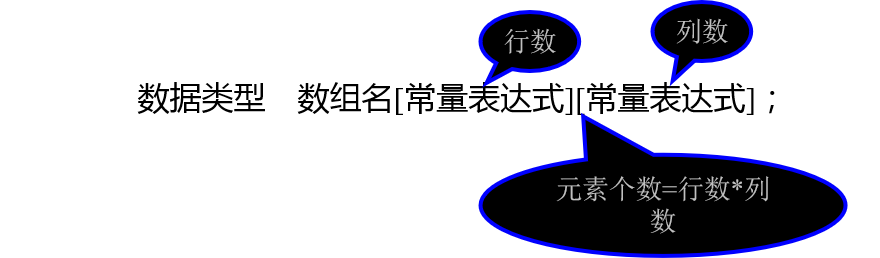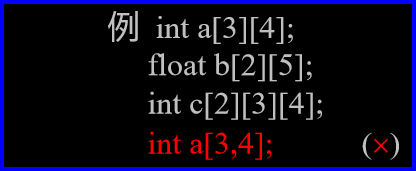数组元素的存放顺序
1、原因： 内存是一维的
2、二维数组： 按行序优先
3、多维数组： 最右下标变化最快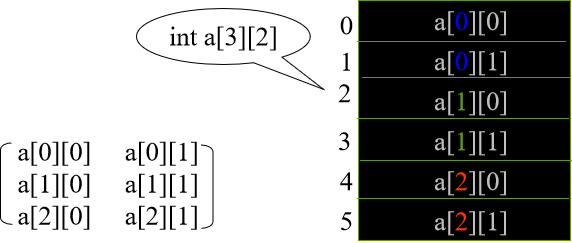数组a[m][n]，m行n列，共m*n个元素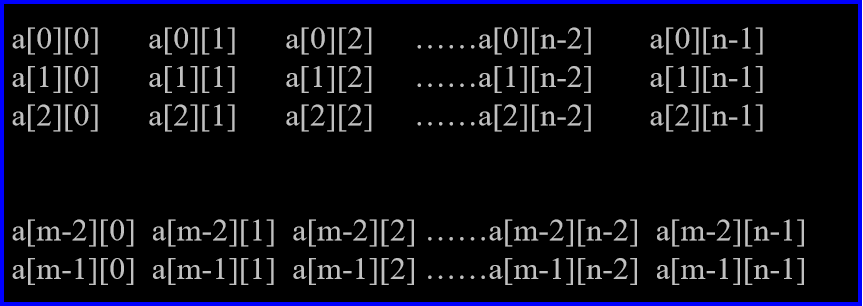这些元素占用m*n个单元，每个单元的大小取决于
元素的类型。
任意元素a[i][j]在数组中的位置计算公式为：
i×n＋j      （从0开始）
a——数组的起始地址；a——0行0列元素的地址。
二维数组元素的引用
形式：  数组名[下标][下标]
二维数组元素的初始化
1、分行初始化
2、按元素排列顺序初始化
程序举例
例1：将二维数组行列元素互换，存到另一个数组中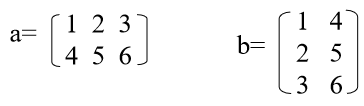/*示例1*/
#include <stdio.h>
main()
{   int a={{1,2,3},{4,5,6}};
int b,i,j;
printf("array a:\n");
for(i=0;i<=1;i++)
{   for(j=0;j<=2;j++)
{   printf("%5d",a[i][j]);
b[j][i]=a[i][j];
}
printf("\n");
}
printf("array b:\n");
for(i=0;i<=2;i++)
{   for(j=0;j<=1;j++)
printf("%5d",b[i][j]);
printf("\n");
}
}

例2：求二维数组中最大元素值及其行列号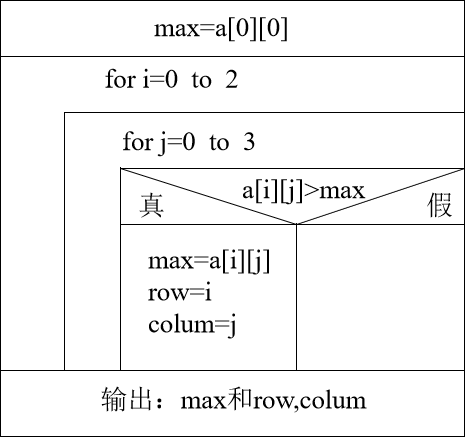/*示例2*/
#include <stdio.h>
main()
{  int a={{1,2,3,4},
{9,8,7,6},
{-10,10,-5,2}};
int i,j,row=0,colum=0,max;
max=a;
for(i=0;i<=2;i++)
for(j=0;j<=3;j++)
if(a[i][j]>max)
{  max=a[i][j];
row=i;
colum=j;
}
printf("max=%d,row=%d,\colum=%d\n",max,row,colum);
}

例3：分别求n阶方阵两条对角线上的元素之和
/*示例3*/
#define N 3
main()
{   int i,j,a[N][N];
int sum1=0,sum2=0;
for(i=0;i<N;i++)
for(j=0;j<N;j++)
scanf(“%d”,&a[i][j]);
for(i=0;i<N;i++)
for(j=0;j<N;j++)
if(i==j) sum1+=a[i][j];
for(i=0;i<N;i++)
for(j=N-1;j>=0;j--)
if((i+j)==N-1) sum2+=a[i][j];
printf(“sum1=%d,sum2=%d\n”,sum1,sum2);
}

字符数组和字符串
字符数组
定义 ： 每个元素存一个字符，主要用来对字符串操作。
字符数组的初始化：
1、逐个字符赋值
2、用字符串常量
字符数组的引用：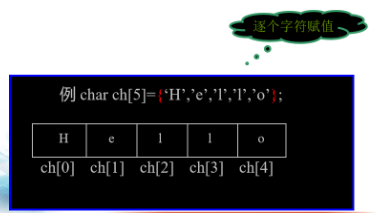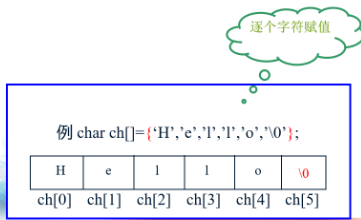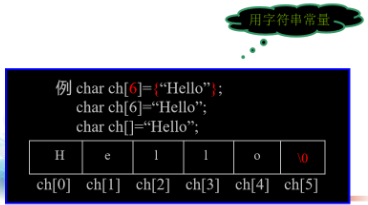字符串
字符串及其结束标志
（1）、无字符串变量，用字符数组处理字符串。
（2）、字符串结束标志：‘\0’。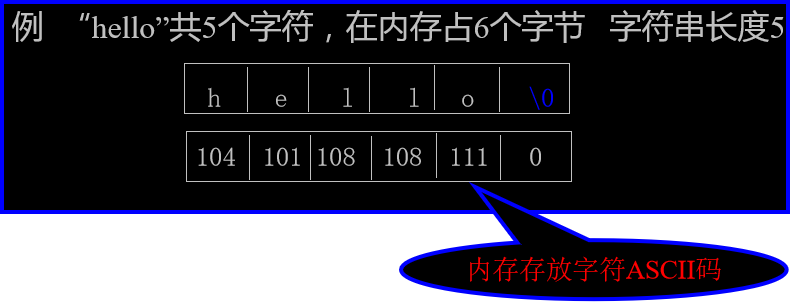字符串的输入输出
输入:

用scanf函数
（1）.输入单个字符：％c  需用循环语句
（2）.输入字符串：%s  scanf(“%s”,str);
用gets函数：gets(str);

输出:

用printf函数
（1）.输出单个字符：％c  需用循环语句
（2）.输出字符串：%s  printf(“%s”,str);
用puts函数：puts(str);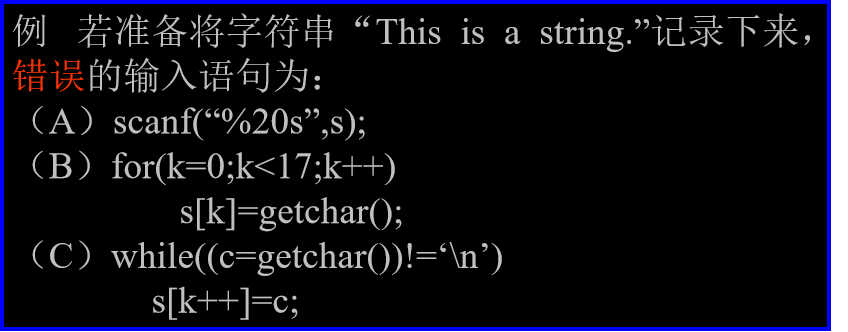常用的字符串处理函数： 加粗样式包含在头文件   string.h
字符串输出函数puts
格式： puts(字符数组)
功能： 向显示器输出字符串（输出完，换行）
说明： 字符数组必须以‘\0’结束
字符串输入函数gets
格式： gets(字符数组)
功能： 从键盘输入一以回车结束的字符串放入字符数组中，并自动加‘\0’
说明： 输入串长度应小于字符数组维数
字符串连接函数strcat
格式： strcat(字符数组1,字符数组2)
功能： 把字符数组2连到字符数组1后面
返值： 返回字符数组1的首地址
说明：
1、字符数组1必须足够大
2、连接前,两串均以‘\0’结束;连接后,串1的‘\0’取消, 新串最后加‘\0’
字符串拷贝函数strcpy
格式： strcpy(字符数组1,字符串2)
功能： 将字符串2，拷贝到字符数组1中去
返值： 返回字符数组1的首地址
说明：
1、字符数组1必须足够大
2、拷贝时‘\0’一同拷贝
3、不能使用赋值语句为一个字符数组赋值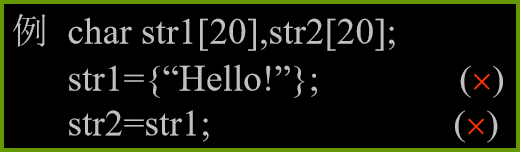字符串比较函数strcmp
格式： strcmp(字符串1,字符串2)
功能： 比较两个字符串
比较规则： 对两串从左向右逐个字符比较（ASCII码），直到遇到不同字符或‘\0’为止
返值： 返回int型整数，
a. 若字符串1< 字符串2， 返回负整数
b. 若字符串1> 字符串2， 返回正整数
c. 若字符串1== 字符串2， 返回零
说明： 字符串比较不能用“==”,必须用strcmp
字符串比较函数strcmp
字符串长度函数strlen
格式：strlen(字符数组)
功能：计算字符串长度
返值：返回字符串实际长度，不包括‘\0’在内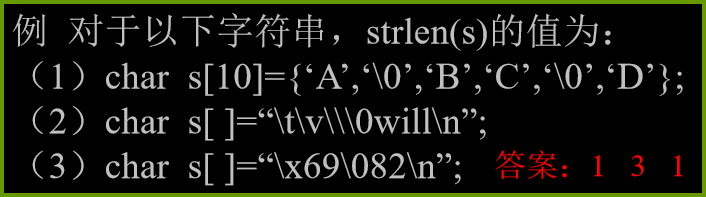展开全文C语言
• C语言总结第七章、数组一维数组一维数组的定义一维数组的引用一维数组的初始化程序举例二维数组及多维数组二维数组的定义二维数组元素的引用二维数组元素的初始化程序举例字符数组和字符串字符数组字符串第七章、...
C语言总结第七章、数组一维数组一维数组的定义一维数组的引用一维数组的初始化程序举例二维数组及多维数组二维数组的定义二维数组元素的引用二维数组元素的初始化程序举例字符数组和字符串字符数组字符串第七章、数组数组是构造数据类型之一数组： 有序数据的集合,用数组名标识元素： 属同一数据类型,用数组名和下标确定一维数组一维数组的定义定义方式：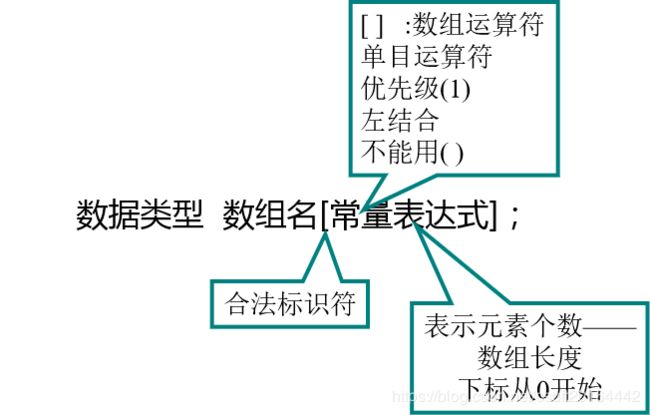例： int a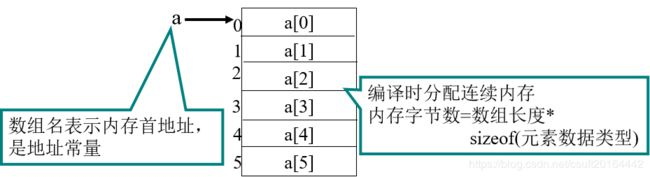一维数组的引用1、数组必须先定义，后使用。2、只能逐个引用数组元素，不能一次引用整个数组。3、数组元素表示形式： 数组名[下标] ,下标可以是常量或整型表达式 。一维数组的初始化初始化方式：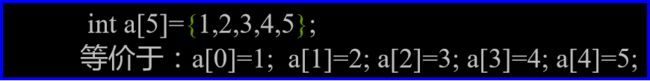说明：1、数组不初始化，其元素值为随机数。2、对static数组元素不赋初值，系统会自动赋以0值。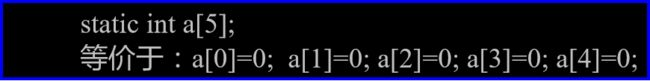3、只给部分数组元素赋初值。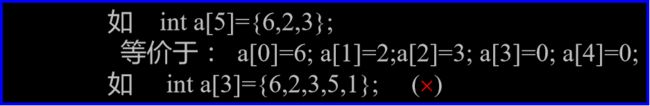4、当全部数组元素赋初值时，可不指定数组长度。程序举例例1： 读10个整数存入数组，找出其中最大值和最小值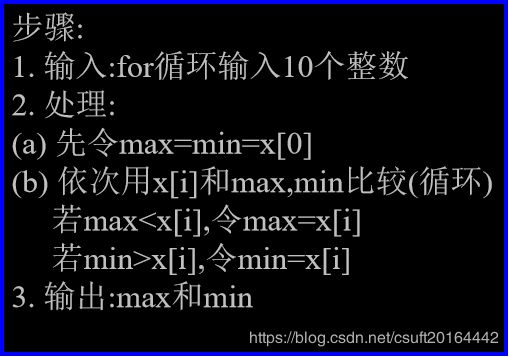/*示例1*/#include#define SIZE 10main(){   int x[SIZE],i,max,min;printf("Enter 10 integers:\n");for(i=0;i{   printf("%d:",i+1);scanf("%d",&x[i]);}max=min=x;for(i=1;i{  if(maxif(min>x[i])  min=x[i];}printf("Maximum value is %d\n",max);printf("Minimum value is %d\n",min);}例2： 用数组求Fibonacci数列前20个数/*示例2*/#includemain(){   int i;int f={1,1};for(i=2;i<20;i++)f[i]=f[i-2]+f[i-1];for(i=0;i<20;i++){   if(i%5==0)  printf("\n");printf("%12d",f[i]);}}例3： 用冒泡法对10个数排序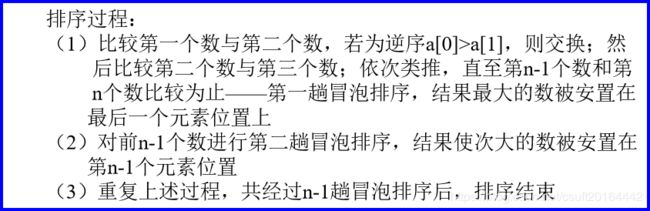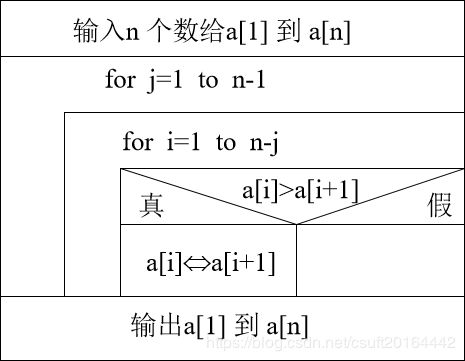/*示例3*/#includemain(){   int a,i,j,t;printf("Input 10 numbers:\n");for(i=1;i<11;i++)scanf("%d",&a[i]);printf("\n");for(j=1;j<=9;j++)for(i=1;i<=10-j;i++)if(a[i]>a[i+1]){t=a[i]; a[i]=a[i+1]; a[i+1]=t;}printf("The sorted numbers:\n");for(i=1;i<11;i++)printf("%d ",a[i]);}例4： 用简单选择法对10个数排序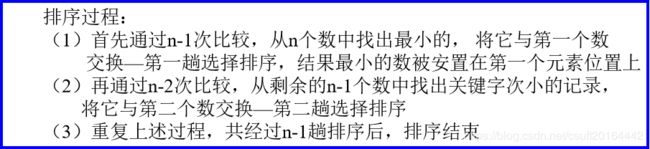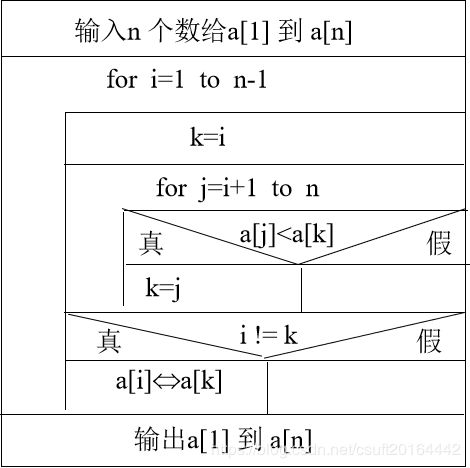/*示例4*/#includemain(){   int a,i,j,k,x;printf("Input 10 numbers:\n");for(i=1;i<11;i++)scanf("%d",&a[i]);printf("\n");for(i=1;i<10;i++){  k=i;for(j=i+1;j<=10;j++)if(a[j]if(i!=k){  x=a[i]; a[i]=a[k]; a[k]=x;}}printf("The sorted numbers:\n");for(i=1;i<11;i++)printf("%d ",a[i]);}二维数组及多维数组二维数组的定义定义方式：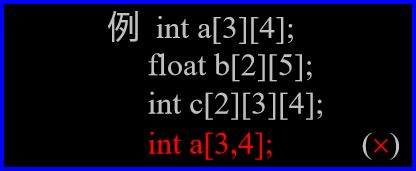数组元素的存放顺序1、原因： 内存是一维的2、二维数组： 按行序优先3、多维数组： 最右下标变化最快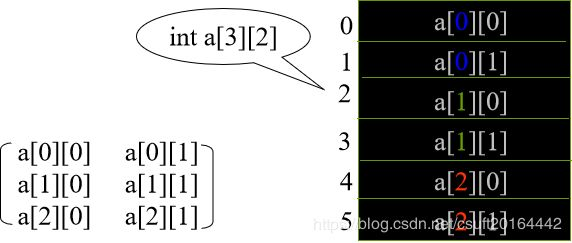数组a[m][n]，m行n列，共m*n个元素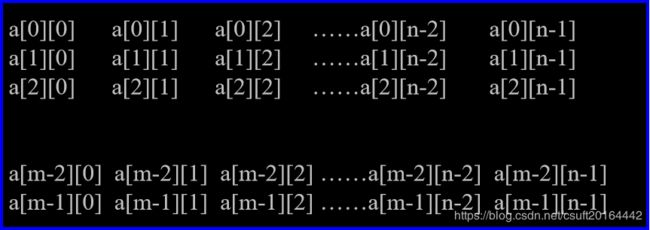这些元素占用m*n个单元，每个单元的大小取决于元素的类型。任意元素a[i][j]在数组中的位置计算公式为：i×n＋j (从0开始)a——数组的起始地址；a——0行0列元素的地址。二维数组元素的引用形式： 数组名[下标][下标]二维数组元素的初始化1、分行初始化2、按元素排列顺序初始化程序举例例1：将二维数组行列元素互换，存到另一个数组中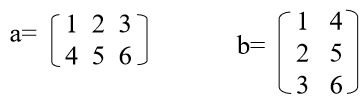/*示例1*/#includemain(){   int a={{1,2,3},{4,5,6}};int b,i,j;printf("array a:\n");for(i=0;i<=1;i++){   for(j=0;j<=2;j++){   printf("%5d",a[i][j]);b[j][i]=a[i][j];}printf("\n");}printf("array b:\n");for(i=0;i<=2;i++){   for(j=0;j<=1;j++)printf("%5d",b[i][j]);printf("\n");}}例2：求二维数组中最大元素值及其行列号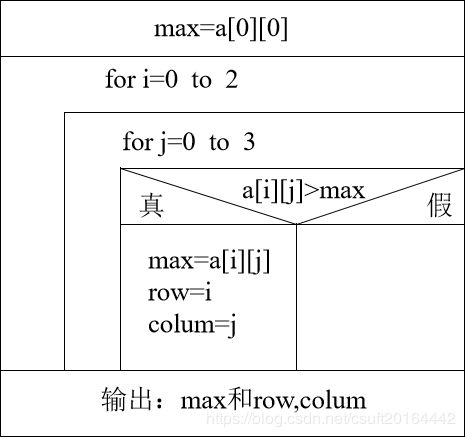/*示例2*/#includemain(){  int a={{1,2,3,4},{9,8,7,6},{-10,10,-5,2}};int i,j,row=0,colum=0,max;max=a;for(i=0;i<=2;i++)for(j=0;j<=3;j++)if(a[i][j]>max){  max=a[i][j];row=i;colum=j;}printf("max=%d,row=%d,\colum=%d\n",max,row,colum);}例3：分别求n阶方阵两条对角线上的元素之和/*示例3*/#define N 3main(){   int i,j,a[N][N];int sum1=0,sum2=0;for(i=0;ifor(j=0;jscanf(“%d”,&a[i][j]);for(i=0;ifor(j=0;jif(i==j) sum1+=a[i][j];for(i=0;ifor(j=N-1;j>=0;j--)if((i+j)==N-1) sum2+=a[i][j];printf(“sum1=%d,sum2=%d\n”,sum1,sum2);}字符数组和字符串字符数组定义 ： 每个元素存一个字符，主要用来对字符串操作。字符数组的初始化：1、逐个字符赋值2、用字符串常量字符数组的引用：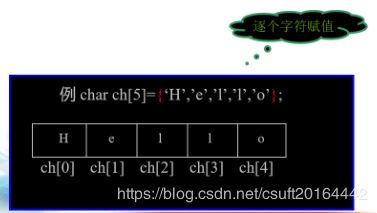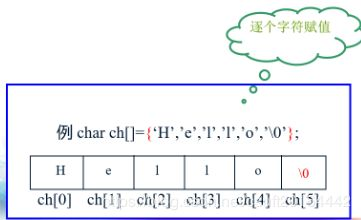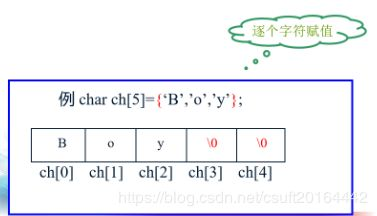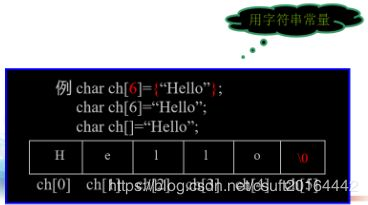字符串字符串及其结束标志(1)、无字符串变量，用字符数组处理字符串。(2)、字符串结束标志：‘\0’。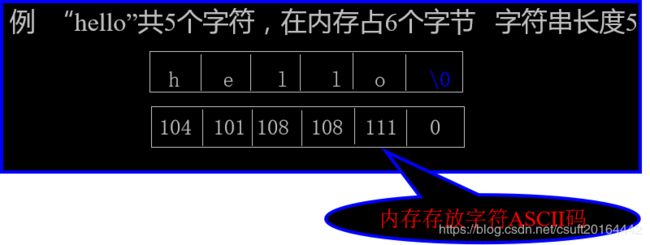字符串的输入输出输入:用scanf函数(1).输入单个字符：％c 需用循环语句(2).输入字符串：%s scanf(“%s”,str);用gets函数：gets(str);输出:用printf函数(1).输出单个字符：％c 需用循环语句(2).输出字符串：%s printf(“%s”,str);用puts函数：puts(str);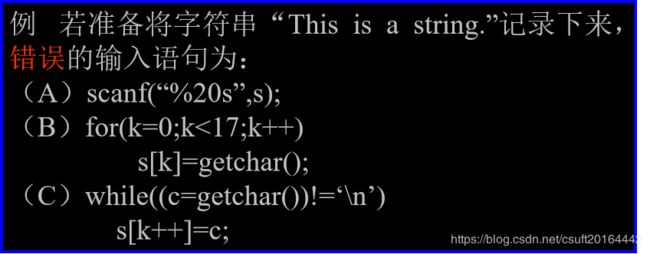常用的字符串处理函数： 加粗样式包含在头文件 string.h字符串输出函数puts格式： puts(字符数组)功能： 向显示器输出字符串(输出完，换行)说明： 字符数组必须以‘\0’结束字符串输入函数gets格式： gets(字符数组)功能： 从键盘输入一以回车结束的字符串放入字符数组中，并自动加‘\0’说明： 输入串长度应小于字符数组维数字符串连接函数strcat格式： strcat(字符数组1,字符数组2)功能： 把字符数组2连到字符数组1后面返值： 返回字符数组1的首地址说明：1、字符数组1必须足够大2、连接前,两串均以‘\0’结束;连接后,串1的‘\0’取消, 新串最后加‘\0’字符串拷贝函数strcpy格式： strcpy(字符数组1,字符串2)功能： 将字符串2，拷贝到字符数组1中去返值： 返回字符数组1的首地址说明：1、字符数组1必须足够大2、拷贝时‘\0’一同拷贝3、不能使用赋值语句为一个字符数组赋值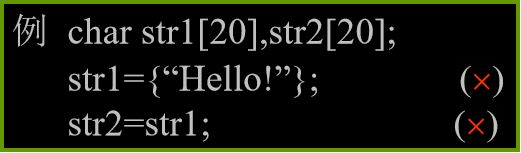字符串比较函数strcmp格式： strcmp(字符串1,字符串2)功能： 比较两个字符串比较规则： 对两串从左向右逐个字符比较(ASCII码)，直到遇到不同字符或‘\0’为止返值： 返回int型整数，a. 若字符串1< 字符串2， 返回负整数b. 若字符串1> 字符串2， 返回正整数c. 若字符串1== 字符串2， 返回零说明： 字符串比较不能用“==”,必须用strcmp字符串比较函数strcmp字符串长度函数strlen格式：strlen(字符数组)功能：计算字符串长度返值：返回字符串实际长度，不包括‘\0’在内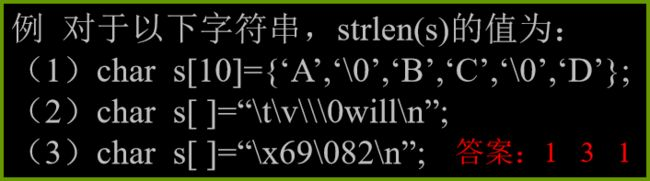展开全文• 一维数组 数组是一种容器，是存储同一种数据类型多个元素集合 1.数组定义 格式1： 数据类型[ ] 数组名 格式2： 数据类型 数组名[ ] 举例： int[ ] a： 定义了一个int类型数组a int a[ ]：定义了一个int类型a...
本章目录一维数组的定义一维数组的初始化一维数组图解一维数组间地址的赋值图解一维数组中常见的操作1、遍历2、获取最值3、反转4、查找指定元素第一次在数组中出现的索引Java 中栈和堆的区别数组操作常见的两个错误二维数组的定义二维数组图解二维数组的遍历杨辉三角递归（5 的阶乘、斐波那契数列）
一维数组的定义
数组：存储同一种数据类型多个元素的集合。既可以存储基本数据类型，也可以存储引用数据类型。
一维数组的定义格式：
格式一：数据类型[] 数组名;       例如：int[] arr;
格式二：数据类型 数组名[];       例如：int arr[];
推荐使用第一种定义格式。
一维数组的初始化
数组的初始化就是为数组中的元素分配内存空间，并为每个元素赋值。只有经过了初始化，数组才能使用。
1、数组初始化的分类：
静态初始化：给出初始化值，由系统决定数组的长度。
动态初始化：给出数组长度，由系统初始化元素的值。
2、初始化的格式：
静态初始化：数据类型[] 数组名 = new 数据类型[]{元素1，元素2，……};        例如：int[] arr = new int[]{1,2,3};
​		       简写：数据类型[] 数组名 = {元素1,元素2,…};     例如：int[] arr = {1,2,3};
动态初始化：数据类型[] 数组名 = new 数据类型[数组长度];       例如：int[] arr = new int;
数组的长度就是数组中元素的个数。
一维数组图解一维数组间地址的赋值图解
定义三个数组，将第一个数组的地址赋给第三个数组，改变第三个数组中的元素后，输出一 三数组中的元素，发现第一数组中的元素也发生了变化。
这是因为，第三数组指向了第一数组的地址，改变第三数组中的元素，实际上是对第一数组中的元素进行了改变。一维数组中常见的操作
1、遍历
public class ArrayDemo02 {
public static void main(String[] args) {
int[] arr = new int[]{1,2,3};
new ArrayDemo02().print(arr);
}

public void print(int[] arr){
for (int i = 0; i < arr.length; i++) {
System.out.println(arr[i]);
}
}
}

2、获取最值
public class ArrayDemo03 {
public static void main(String[] args) {
int[] ints = new int[]{15,45,-78,213,-98,156};
System.out.println(new ArrayDemo03().getMax(ints));
System.out.println(new ArrayDemo03().getMin(ints));
}

//获取数组中的最大值
public int getMax(int[] arr) {
int tump = arr;
for (int i = 1; i < arr.length; i++) {
if (arr[i] > tump) {
tump = arr[i];
}
}
return tump;
}

//获取数组中的最小值
public int getMin(int[] arr) {
int tump = arr;
for (int i = 1; i < arr.length; i++) {
if (arr[i] < tump) {
tump = arr[i];
}
}
return tump;
}
}

3、反转
public class ArrayDemo04 {
public static void main(String[] args) {
int[] ints = {1, 2, 3, 4, 5};
int[] reverse = new ArrayDemo04().getReverse(ints);
System.out.println(Arrays.toString(reverse));
}

public int[] getReverse(int[] arr){
int[] ints = new int[arr.length];
for (int i = 0; i < arr.length; i++) {
ints[i] = arr[arr.length-1-i];
}
return ints;
}
}

4、查找指定元素第一次在数组中出现的索引
public class ArrayDemo05 {
public static void main(String[] args) {
int[] ints = {1, 2, 3, 4, 3, 8, 6, 4, 8};
System.out.println("结果是："+new ArrayDemo05().getIndex(ints, 4));
}

public String getIndex(int[] ints, int a) {
for (int i = 0; i < ints.length; i++) {
if (ints[i] == a) {
return String.valueOf(i);
}
}
return "数组中没有所查元素！";
}
}

Java 中栈和堆的区别
栈：存放局部变量，局部变量：在方法定义中或方法声明上的变量。
堆：存放创建的对象。
堆的特点：
每一个创建出来的对象，系统都会为它分配一个地址值。
​每一个变量都有初始化值，byte、short、int、long 的默认值为 0；float、double 的默认值为 0.0；char    	   的默认值为 ‘ ’（\u0000）；boolean 的默认值是 false；引用数据类型的默认值是 null。
​对象使用完毕后就成了垃圾，等待被回收。
数组操作常见的两个错误
ArrayIndexOutOfBoundsException：数组索引越界异常，原因是访问了不存在的索引。
NullPointerException：空指针异常，原因是数组已不再指向堆内存，我们还用数组访问元素。
二维数组的定义
二维数组：每个元素都是一维数组的数组。
二维数组的定义格式：
格式一：
数据类型[][] 数组名 = new 数据类型[m][n];
格式一有两种变型，但不推荐使用：
数据类型 数组名[][] = new 数据类型[m][n];
数据类型[] 数组名[] = new 数据类型[m][n];
说明：m 表示这个二维数组有多少个一维数组，必须给出；n 表示每个一维数组中元素的个数，可以省略。
例如：int[][] arr = new int，表示：定义了一个二维数组，这个二维数组中有三个一维数组，分别是：arr、arr、arr；每个一维数组中都有两个元素，可以通过 arr[m][n] 来获取。
格式二：
数据类型[][] 数组名 = new 数据类型[m][];
说明：m 表示这个二维数组中有多少个一维数组，没有给出每个一维数组中元素的个数，可以动态给出。
例如：int[][] arr = new int[];
​	              arr = new int;
​	              arr = new int;
​	              arr = new int;
表示：定义一个二维数组，这个二维数组中有三个一维数组，分别是：arr、arr、arr；arr 中有两个元素、arr 中有三个元素、arr 中有一个元素。
格式三
数据类型[][] 数组名 = new 数据类型[m][n]{{元素1,…},{元素1,元素2,…},{元素1,元素2,元素3,…},…};
简写：数据类型[][] 数组名 = {{元素1,…},{元素1,元素2,…},{元素1,元素2,元素3,…},…};
例如：int[][] arr = new int{{1,2},{1,2},{1,2}};
​	            int[][] arr = {{1,2},{1,2},{1,2}};
二维数组图解
格式一图解：格式二图解：格式三图解：二维数组的遍历
public class ArrayDemo06 {
public static void main(String[] args) {
int[][] ints = {{1, 2}, {3,4}, {5, 6}};
new ArrayDemo06().print(ints);
}

public void print(int[][] ints){
for (int i = 0; i < ints.length; i++) {
for (int j = 0; j < ints[i].length; j++) {
System.out.println(ints[i][j]);
}
}
}
}

杨辉三角
打印杨辉三角形，行数键盘录入
需求：打印杨辉三角
1
1	1
1	2	1
1	3	3	1
1	4	6	4	1
1	5	10	10	5	1

public class ArrayDemo07 {
public static void main(String[] args) {
Scanner scanner = new Scanner(System.in);
System.out.println("请输入杨辉三角行数：");
int a = scanner.nextInt();

int[][] print = new ArrayDemo07().print(a);
for (int[] ints1 : print) {
for (int i : ints1) {
System.out.print(i+"\t");
}
System.out.println();
}
}

public int[][] print(int a){
//二维数组的第一列和最后一列都是1
/**
* 杨辉三角的形状是一个三角形，所以创建二维数组时的行列不能相同，相同创建成了正方形
* 所以，创建二维数组时，只给定行数，列数根据需要再进行创建
* 这时，创建的列数为：行数 + 1，因为行从 0 开始记
* 每一行的第一个值为 0
* 每一行的最后一个值为 0
*/
int[][] ints = new int[a][];
for (int i = 0; i < a; i++) {
//列数 = 行数 + 1
ints[i] = new int[i+1];
//每一行的第一个值为 0
ints[i] = 1;
//每一行的最后一个值为 0
ints[i][i] = 1;
}

/**
* 从第三行开始，除去每行的第一、最后一个数，其余数的值 = 上面的数 + 左上方的数
* 也就是 本列的上一行数 + 前一列上一行数
* 从第三行开始，直到最后一行都需要对其余的数进行计算
* 从第三行第二个数开始后，直到最后一行的倒数第二个数，都要进行计算
*/
//i = 2，是从第三行
for (int i = 2; i < a; i++) {
// j = 1，是第二个数，ints[i].length-1，是该行的倒数第二个数
for (int j = 1; j < ints[i].length-1; j++) {
ints[i][j] = ints[i-1][j] + ints[i-1][j-1];
}
}

return ints;
}
}

递归（5 的阶乘、斐波那契数列）
程序调用自身的编程技巧叫做递归，它通常把一个大型复杂的问题层层转化为一个与原问题相似的规模较小的问题来求解，递归只需少量的程序就可描述出解题过程所需要的多次重复计算，极大地减少了程序的代码量。
求 5 的阶乘
图解：public class RecursionDemo01 {
public static void main(String[] args) {
System.out.println(new RecursionDemo01().getRecursion(5));
}

public int getRecursion(int a) {
if (a == 1) {
return 1;
} else {
return a * getRecursion(a - 1);
}
}
}

斐波那契数列
1 , 1 , 2 , 3 , 5 , 8 …求第 40 位的值。
从第3位数开始，本位数是前两位数之和。要计算第多少位的值，那么就需要将位数作为参数传进方法进行计算。
public class RecursionDemo02 {
public static void main(String[] args) {
System.out.println(new RecursionDemo02().getRecursion(40));
}

public int getRecursion(int a) {
if (a == 1 || a == 2) {
return 1;
} else {
return getRecursion(a - 1) + getRecursion(a - 2);
}
}
}


真正要做的事，对神明都不会讲.
各位看官本章结束，我们下篇再见 O(∩_∩)O~
-Czx.


展开全文java
• 该方法不会改变现有的数组，而仅仅会返回被连接数组的一个副本。 举例说明： /*concat()结果返回数组*/ var arr = new Array(3) arr = "George" arr = "John" arr = "Thomas" var ar....

首先：concat方法
定义：
concat() 方法用于连接两个或多个数组。该方法不会改变现有的数组，而仅仅会返回被连接数组的一个副本。
举例说明：

/*concat()结果返回的是一个数组*/

var arr = new Array(3)
arr = "George"
arr = "John"
arr = "Thomas"

var arr2 = new Array(3)
arr2 = "James"
arr2 = "Adrew"
arr2 = "Martin"

document.write(arr.concat(arr2))

//result:
George,John,Thomas,James,Adrew,Martin


join方法：
join() 方法用于把数组中的所有元素放入一个字符串。元素是通过指定的分隔符进行分隔的。
举例说明：

/*join()返回的是一个字符串*/
var arr = new Array(3);
arr = "George"
arr = "John"
arr = "Thomas"

document.write(arr.join());

document.write("<br />");

document.write(arr.join("."));
//result:
George,John,Thomas
George.John.Thomas


slice方法：
返回一个数组的一段。（仍为数组） arrayObj.slice(start, [end])  参数 arrayObj  必选项。一个 Array 对象。  start  必选项。arrayObj 中所指定的部分的开始元素是从零开始计算的下标。  end  可选项。arrayObj 中所指定的部分的结束元素是从零开始计算的下标。  说明 slice 方法返回一个 Array 对象，其中包含了 arrayObj 的指定部分。 slice 方法一直复制到 end 所指定的元素，但是不包括该元素。如果 start 为负，将它作为 length + start处理，此处 length 为数组的长度。如果 end 为负，就将它作为 length + end 处理，此处 length 为数组的长度。如果省略 end ，那么 slice 方法将一直复制到 arrayObj 的结尾。如果 end 出现在 start 之前，不复制任何元素到新数组中。 示例 在下面这个例子中，除了最后一个元素之外，myArray 中所有的元素都被复制到 newArray 中：  newArray = myArray.slice(0, -1)
二维数组的定义方法：
方式一：var arr = [[a,b,c,d],[a1,b1,c1,d1],[a2,b2,c2,d2]],
方式二:  var arr = new Array([a,b,c,d],[a1,b1,c1,d1],[a2,b2,c2,d2]),

转载于:https://www.cnblogs.com/ourLifes/p/7884592.html
展开全文• 一维数组 数组概念和定义格式 数组概念 数组是存储同一种数据类型多个元素集合。也可以看成是一个容器。 数组既可以存储基本数据类型，也可以存储引用数据类型 数组定义格式 格式1： 数据类型[] 数组名； 格式2：...java
• 该方法不会改变现有的数组，而仅仅会返回被连接数组的一个副本。 举例说明： 1 /*concat()结果返回数组*/ 2 3 var arr = new Array(3) 4 arr = "George" 5 arr = "John" 6 arr = ...
• （这个定义可能有点片面，但是二维数组的原理大致是这样，在很多语言中，对于二维数组已经三维数组，或者说是多为数组，都是这种思想，比如c语言，c++等，只不过在不同的语言中，可能定义方式有些出入）。...
• //第二种定义维数组的方式，它的行数确定，但是每行的列数不确定。这样的的数组实现方法：先创建制定行数，列数缺省的二维数组，然后对数组的每行重新初始化。 public class TwoArrayDem...
• 数组概述和定义格式说明 1.数组概念 数组是存储同种数据类型多个元素的集合。也可以看成是个容器。 数组既可以存储基本数据类型，也可以存储引用数据类型。...推荐使用第定义方式数组的初始化动态初...Java基础知识 二维数组
• 一.一维数组 （一）一维数组定义格式 ...（二）一维数组的初始化 Java中的数组必须先初始化,然后才能使用 （1）初始化的分类： a:动态初始化: 只指定长度，由系统给出初始化值 b:静态初始化...
• /* * 维数组的定义方式： * 第种：数据类型[] 变量名 = new 数据类型[长度]; * 第二种：数据类型[] 变量名名 = {元素，元素，元素}; * 第三种：数据类型[] 变量名 = new 数据类型[]{元素，元素...}; * */ 举例 ...
• 一维数组格式: ​ 格式1： 数据类型[] 数组名； ​ 格式2： 数据类型 数组名[]; 举例： ​ int[] a; 定义了一个int类型的数组a； ​ int a[]; 定义了一个int类型的a数组； ​ 一般使用第一种定义方式数组的初始化...Java
• 维数组的定义 方式一：元素类型[][] 数组名称； 方式二：元素类型 数组名称[][]； 推荐使用方式一 数组的初始化 静态初始化： 语法：元素类型[][] 数组名称 = new 元素类型[][]{{一维数组1,一维数组2,一维...
• 维数组的定义 方式一：元素类型[][] 数组名称； 方式二：元素类型 数组名称[][]； 推荐使用方式一 数组的初始化 静态初始化： 语法：元素类型[][] 数组名称 = new 元素类型[][]{{一维数组1,一维数组2,一维...
• 维数组的 定义格式： 数组类型[][] 变量名 = new 数据类型[长度1][长度2]; 长度1：条烟有多少盒 长度2：盒烟有多少根 二维数组的 初始化方式： 动态初始化： 数据类型[][] 变量名 = ne...
• 一维数组的初始化方法 方式一：静态初始化 数据类型[] 数组名 = {元素1,元素2,元素3...};//必须在一个语句中完成，不能分开两个语句写 举例定义存储1，2，3，4，5整数的数组容器 int[] arr = {1,2,3,...java
• 11_Java筑基之二维数组 1. 二维数组的概念 本质上还是一个一维数组,只是其数组元素又是一个一维数组. 举例说明: 变量,一维数组,二维数组...2. 二维数组的定义 方式一: 元素类型[][] 数组名称; 方式二: 元...
• 数组的定义格式: 数组的定义格式: 方式1) 数据类型[] 数组名称 ; (推荐) 举例: int[] arr ; 定义了个int类型的数组arr变量 方式2) 数据类型 数组名称[] ; 举例 int arr[]...java
• STL之vector用法 ...可以有五种方式,举例说明如下： （1） vector<int> a(10);//定义了10个整型元素向量（尖括号中为元素类型名，它可以是任何合法数据类型），但没有给出初值，其值是不确数据结构 stl
• 数组的特点 ...数组的定义格式1、数组类型[] 数组名；2、数组类型 数组名[]； 推荐使用方式1数组的初始化1、动态初始化（只给长度，系统默认给初始值）举例：int[] arr = new int;2、静态初始化java 内存分配 存储 内存
• 1、详细写出数组定义的几种方式，并举例说明 定义数组：  1.动态初始化  数据类型 [] 变量名=new 数据类型[容量];   例如：定义一个int类型数组 分配5个元素  int [] arr=new int;  2.静态初始化  数据...
• 一维数组定格式 格式1： 数据类型[] 数组名； 格式2： 数据类型 数组名[]; 举例： int[] a； 定义了一个int类型的数组a； int a[]; 定义了一个int类型的a数组；...n表示每一个一维数组的元素个数 可...
• 1、一维数组的定义方式 // 类型说明符 数组名 [常量表达式]； // 2、举例 // int a; 说明整型数组 a，有 10 个元素。 float b,c; 说明实型数组 b，有 10 个元素，实型数组 c，有 20 个元素。 // 3...
• 维数组可以看成以数组为元素数组。 其实多维数组不仅仅只有二维数组,还有三维数组,四维数组..., 但是三维以上很少见到,所以大家重点了解二维数组即可。 举例 int [][] a = {{1,2},{3,4,0,9},{5,6,7}}; ...字符串 java 数据结构 leetcode 编程语言
• .稀疏数组sparsearray 定义：当个数组元素中大部分元素为0，或者为同一值时，可使用稀疏数组保存数组。即从原数组中抽取有效...因为该二维数组的很多值是默认值0，因此记录了很多没有意义的数据->稀疏数组，12算法 数据结构 java
• ## （谭版）7 数组

千次阅读 2006-10-27 18:46:00
7 数组... 17.1 一维数组的定义和引用..... 17.1.1 一维数组的定义方式... 17.1.2 一维数组元素的引用... 27.1.3 一维数组的初始化... 47.1.4 一维数组程序举例... 47.2 二维数组的定义和引用... 67.2.1 二维数语言 input c string